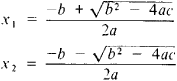# Quadratic Equation

(redirected from ABC formula)
Also found in: Dictionary, Thesaurus.
Related to ABC formula: quadratic formula

## quadratic equation

[kwä′drad·ik i′kwā·zhən]
(mathematics)
Any second-degree polynomial equation.
McGraw-Hill Dictionary of Scientific & Technical Terms, 6E, Copyright © 2003 by The McGraw-Hill Companies, Inc.
The following article is from The Great Soviet Encyclopedia (1979). It might be outdated or ideologically biased.

## Quadratic Equation

an equation of the form ax2 + bx + c = 0, where a, b, and c are any number and are called the coefficients of the equation. A quadratic equation has two roots, which are found by the formulasThe expression D = b2 − 4ac is called the discriminant of the quadratic equation. If D > 0, then the roots of the quadratic equation are real and unequal; if D < 0, then the roots areconjugate complex numbers; if D = 0, then the roots are real andequal. The Vièta formulas x1 + x2 = − b/a and x\x2 = c/a link the roots and coefficients of a quadratic equation. The left-hand side of a quadratic equation can be expressed in the form α (xx2) (xx2). The function y = ax2 + bx + c is called a quadratic trinomial, and its graph is a parabola with the vertex at the point M (−b/2a; cb2/4a) and axis of symmetry parallel to the. y-axis; the direction of the branches of the parabola coincides with the sign of a. The solution of the quadratic equation was already known in geometric form to ancient mathematicians.

The Great Soviet Encyclopedia, 3rd Edition (1970-1979). © 2010 The Gale Group, Inc. All rights reserved.
Site: Follow: Share:
Open / Close